# Texas Go Math Grade 1 Lesson 14.5 Answer Key Create New Two-Dimensional Shapes

Refer to our Texas Go Math Grade 1 Answer Key Pdf to score good marks in the exams. Test yourself by practicing the problems from Texas Go Math Grade 1 Lesson 14.5 Answer Key Create New Two-Dimensional Shapes.

## Texas Go Math Grade 1 Lesson 14.5 Answer Key Create New Two-Dimensional Shapes

Unlock the Problem

Cora wants to combine shapes to make a circle. She has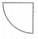. How can Cora make a circle?
Answer:  Cora has one arc. She wants to combine another three arcs to make the whole circle.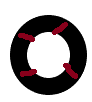What information am I given?

Cora uses this shape. :Plan

What is my plan or strategy?

I can combine the arcs which are used by Cora and make a circle.

Solve

Show how to solve the problem.

STEP 1:
Use shapes. Combine to make a new shape.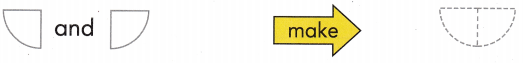STEP 2:
Then use the new shape.flipping on another side we can get another shape.
STEP 2:
I use moon shape to complete the circle.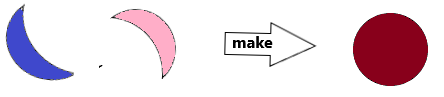HOME CONNECTION • Recognizing how shapes con be put together and taken apart provides o foundation for future work with fractions.
Answer: Make children do activities by giving home appliances which are in shapes like circle, triangle, square, rectangle etc. And try to combine and make new shapes.

Try Another Problem

Use shapes to solve. Draw to show your workQuestion 1.
Useto make a larger.
STEP 1:
Combine shapes to make a new shape.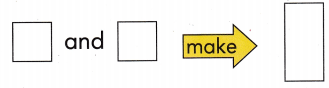STEP 2:
Then use the new shape.Answer: For step 2 we use two triangles to get a square.Question 2.
Useto make a.
STEP 1:
Combine shapes to make a new shape.STEP 2:
Then use the new shape.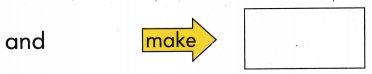Answer: Two right-angle triangles are used to make rectangle.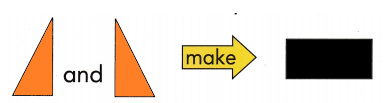Math Talk
Mathematical Processes

Describe how you made the rectangle in Exercise 2.
Answer: I use two triangles to make a rectangle.

Share and Show

Use shapes to solve. Draw to show your work.

Question 3.
Use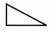to make a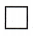.
STEP 1:
Combine shapes to make a new shape.STEP 2:
Then use the new shape.Answer: We can use triangles to make a square.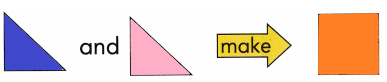Problem Solving

Question 4.
H.O.T. Multi-Step
Use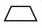andto make a.STEP 1:
Combine shapes to make a new shape.STEP 2:
Then use the new shape.Answer: I use trapezium and triangle to get a parallelogram.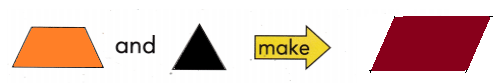Question 5.
Reasoning Which two shapes make a circle?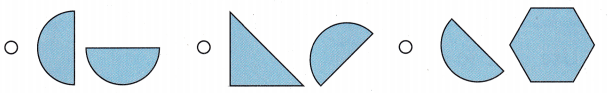Answer: I use two semi-circles to make a whole circle.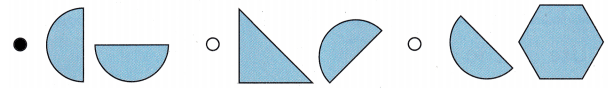Question 6.
Which two shapes make a triangle?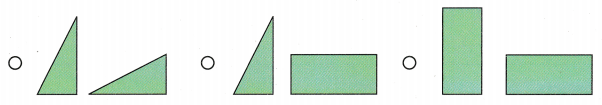Answer: The first one is correct.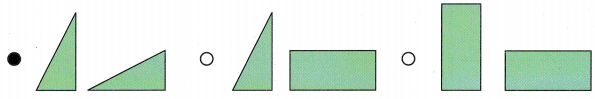Question 7.
Multi-Step Gabe uses pattern blocks to make a new shape. He uses 1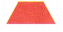and 3. Which of these shapes can he make?
(A) circle
(B) square
(C) hexagon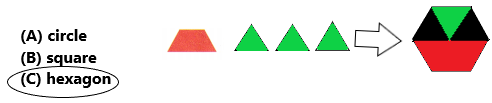The total explanation is represented in the above diagram.

Question 8.
Texas Test Prep Which new shape could you make?TAKE HOME ACTIVITY • Have your child explain how he or she solved Exercise 6.

### Texas Go Math Grade 1 Lesson 14.5 Homework and Practice Answer Key

Use shapes to solve. Draw to show your work.

Question 1.
Use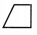to make a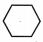.
STEP 1:
Combine shapes to make a new shape.STEP 2:
Then use the new shape.Answer: Two trapeziums are needed to get a hexagon.Problem Solving

Question 2.
Multi-Step Useandto make a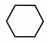.
STEP 1:
Combine shapes to make a new shape.STEP 2:
Then use the new shape.
andAnswer: One triangle and one rhombus are used to get a hexagon.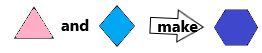Lesson Check

Question 3.
Which two shapes make a rectangle?Answer: Two squares can make a rectangle.Question 4.
Nikki makes a star. She uses onlyand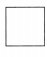. Which star does she make?Answer: The second option is correct.Question 5.
Multi-Step Jeanne uses pattern blocks to make a new shape. She uses 4and 1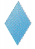. Which new shape does she make?Answer: The second option is correct.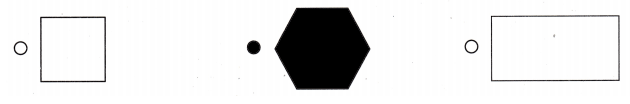Scroll to Top